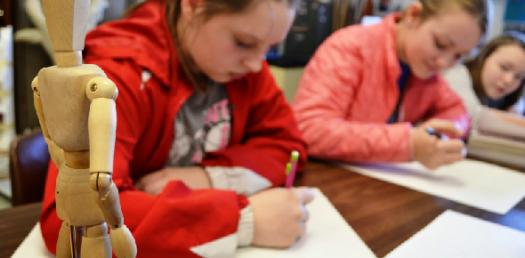# 7th Grade Quiz On Design Process

10 Questions | Total Attempts: 770SettingsIn design class, we get to put our ideas into concrete, audio, or visual form. Not only does this class improve your creativeness, but it shows you just how attainable your vision or imagination is. As a seventh-grader, how much have you understood the design process so far and the steps? Take up the quiz below and get to find out!

Related Topics
• 1.
• 2.
(True or False) Even if you are not going to be an inventor or engineer, learning how to think like one is good for EVERYONE!
• A.

True

• B.

False

• 3.
What is the 6th step of the design process?
• A.

Select and refine the best solution

• B.

Develop potential solutions

• C.

Gather Information

• D.

Build a prototype

• E.

Test the solution

• F.

Identify the problem or need

• 4.
What is the 5th step of the design process?
• A.

Select and refine the best solution

• B.

Develop potential solutions

• C.

Gather Information

• D.

Build a prototype

• E.

Test the solution

• F.

Identify the problem or need

• 5.
What is the 4th step of the design process?
• A.

Select and refine the best solution

• B.

Develop potential solutions

• C.

Gather Information

• D.

Build a prototype

• E.

Test the solution

• F.

Identify the problem or need

• 6.
What is the 3rd step of the design process?
• A.

Select and refine the best solution

• B.

Develop potential solutions

• C.

Gather Information

• D.

Build a prototype

• E.

Test the solution

• F.

Identify the problem or need

• 7.
What is the 2nd step of the design process?
• A.

Select and refine the best solution

• B.

Develop potential solutions

• C.

Gather Information

• D.

Build a prototype

• E.

Test the solution

• F.

Identify the problem or need

• 8.
What is the 1st step of the design process?
• A.

Select and refine the best solution

• B.

Develop potential solutions

• C.

Gather Information

• D.

Build a prototype

• E.

Test the solution

• F.

Identify the problem or need

• 9.
The 1st step of the design process is to Identify the problem or the_______
• A.

Solution

• B.

Opportunity

• C.

Need

• D.

Potential

• 10.
What is the KEY INGREDIENT to being a successful inventor and problem-solver?
• A.

Genius

• B.

Persistence

• C.

Math skills

• D.

Energy